MOBILE MATH – Math on the Go
www.mobilemath.ca

# 3 - Numeracy - Dividing

Summary – Examples Covered

Screen Shot

3.1  – Numeracy – Introduction to Division

Smart Notebook Lesson 3.1

Learning Goal:

By the end of this lesson I should be able to DIVIDE small positive numbers, WITHOUT the use of a calculator (technology).

Techniques:    Picture Model,       Area Model,       Number Model

Examples:

(a)               18 ÷ 3

(b)              24 ÷ 8

(c)               16 ÷ 23.2 – Numeracy – Dividing using Group Subtraction

Smart Notebook Lesson 3.2

Learning Goal:

By the end of this lesson I should be able to DIVIDE positive numbers using a group subtraction model, WITHOUT the use of a calculator (technology).

Techniques:    Group Subtraction (Dutch, Continental, European Division)

Examples:

(a)               16 ÷ 2

(b)              32 ÷ 4

(c)               128 ÷ 8

(d)               308 ÷ 14

(e)               1984 ÷ 124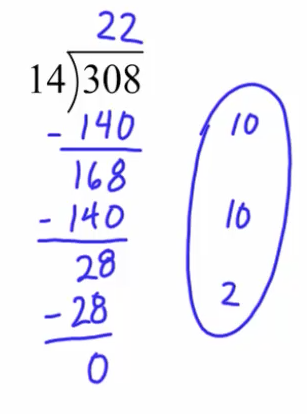3.3  – Numeracy – Dividing using Long Division

Smart Notebook Lesson 3.3

Learning Goal:

By the end of this lesson I should be able to DIVIDE positive numbers using LONG Division, WITHOUT the use of a calculator (technology)..

Techniques:    Group Subtraction, Long Division

Examples:

(a)               52 ÷ 4

(b)              128 ÷ 8

(c)               308 ÷ 14

(d)               1984 ÷ 124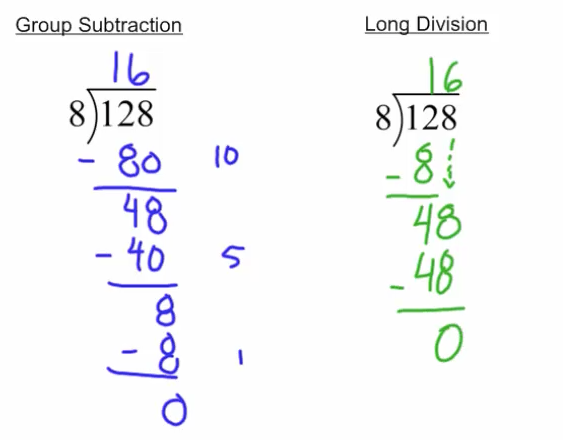3.4  – Numeracy – Dividing Large Numbers using Group Subtraction or Long Division

Smart Notebook Lesson 3.4

Learning Goal:

By the end of this lesson I should be able to DIVIDE large positive numbers, WITHOUT the use of a calculator (technology).

Techniques:    Group Subtraction, Long Division

Examples:

(a)               1012 ÷ 44

(b)              960 ÷ 15

(c)               2844 ÷ 18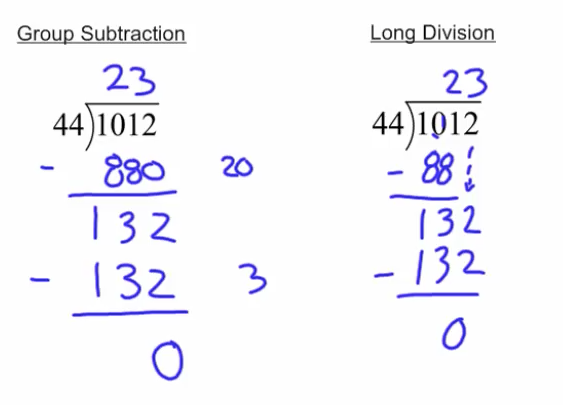3.5  – Numeracy – Division with a Remainder

Smart Notebook Lesson 3.5

Learning Goal:

By the end of this lesson I should be able to DIVIDE positive numbers that have a Remainder, WITHOUT the use of a calculator (technology).

Techniques:  Group Subtraction, Long Division

Examples:

(a)               9 ÷ 2

(b)              16 ÷ 5

(c)               19 ÷ 6

(d)              26 ÷ 11

(c)               191 ÷ 143.6  – Numeracy – Dividing by a Decimal Number

Smart Notebook Lesson 3.6

Learning Goal:

By the end of this lesson I should be able to DIVIDE whole numbers by a decimal number, WITHOUT the use of a calculator (technology).

Techniques:   Group Subtraction, Long Division

Examples:

(a)               10 ÷ 2.5

(b)              17 ÷ 4.25

(c)               25 ÷ 3.1253.7  – Numeracy – Dividing a Decimal Number by a Decimal Number

Smart Notebook Lesson 3.7

Learning Goal:

By the end of this lesson I should be able to DIVIDE a decimal number by another decimal number, WITHOUT the use of a calculator (technology).

Techniques:  Group Subtraction, Long Division

Examples:

(a)               17.5 ÷ 2.5

(b)              28.5 ÷ 1.5

(c)               24.9 ÷ 4.15

(d)              21.875 ÷ 3.125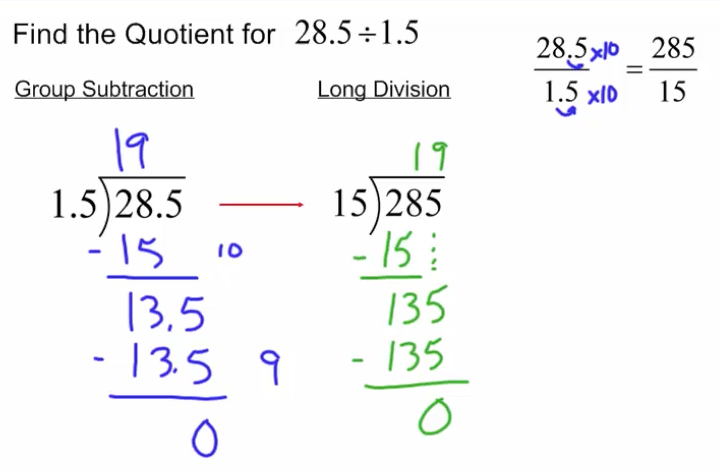3.8  – Numeracy - Dividing a Decimal humber by a Decimal Number with a Remainder

Smart Notebook Lesson 3.8

Learning Goal:

By the end of this lesson I should be able to DIVIDE a decimal number by another decimal number and get a result that has a remainder, WITHOUT the use of a calculator (technology).

Techniques:  Group Subtraction, Long Division

Examples:

(a)               28.5 ÷ 1.6

(b)              24.9 ÷ 4.5

(c)               20.8 ÷ 3.125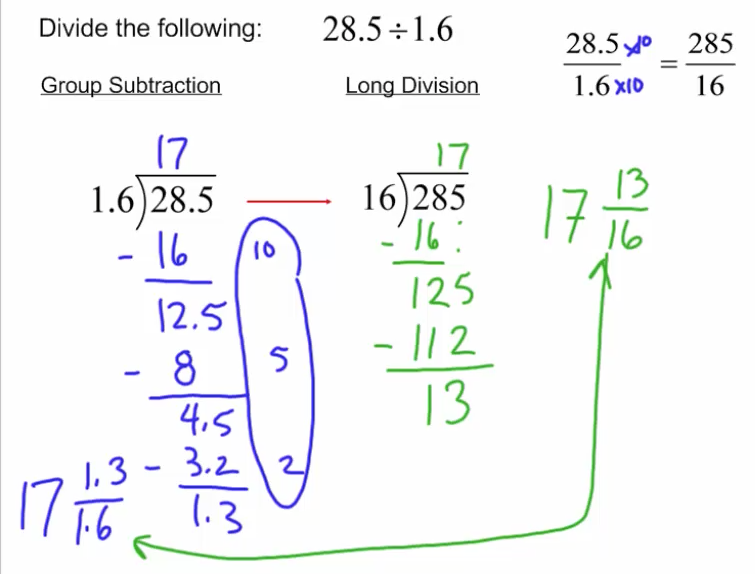3.9  – Numeracy – Dividing by a Decimal Number

Smart Notebook Lesson 3.9

Learning Goal:

By the end of this lesson I should be able to DIVIDE positive and negative numbers together, WITHOUT the use of a calculator (technology).

Techniques:  Group Subtraction, Long Division

Examples:

(a)               -8 ÷ 2

(b)              (-8) ÷ (-2)

(c)               (-60) ÷ 15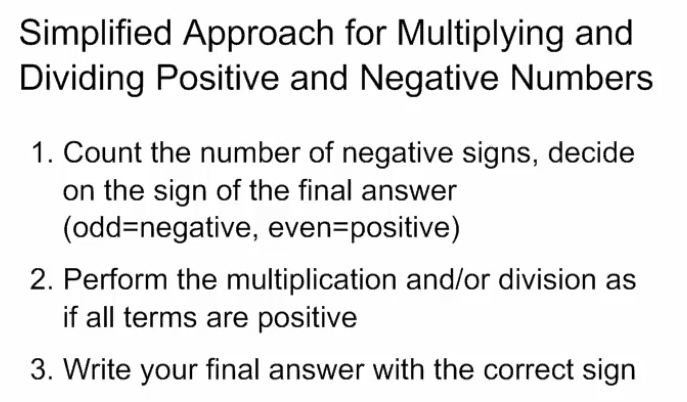3.10  – 3.11 - Numeracy – Dividing Review and Final Assessment

Task 3.10 – Review - Word

Task 3.10 - Review - Pdf

Final Assessment 3.11 - Word

Final Assessment 3.11 - Pdf

Summative Activities for the Dividing Unit